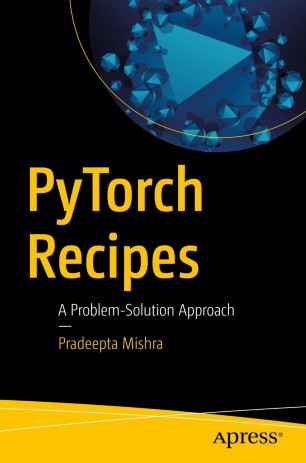# PyTorch Recipes

## A Problem-Solution ApproachBook

1. Front Matter
Pages i-xx
Pages 1-27
Pages 29-48
Pages 49-109
Pages 111-126
Pages 127-149
Pages 151-164
Pages 165-178
9. Back Matter
Pages 179-184

### Introduction

Get up to speed with the deep learning concepts of Pytorch using a problem-solution approach. Starting with an introduction to PyTorch, you'll get familiarized with tensors, a type of data structure used to calculate arithmetic operations and also learn how they operate. You will then take a look at probability distributions using PyTorch and get acquainted with its concepts. Further you will dive into transformations and graph computations with PyTorch. Along the way you will take a look at common issues faced with neural network implementation and tensor differentiation, and get the best solutions for them.

Moving on to algorithms; you will learn how PyTorch works with supervised and unsupervised algorithms. You will see how convolutional neural networks, deep neural networks, and recurrent neural networks work using PyTorch. In conclusion you will get acquainted with natural language processing and text processing using PyTorch.

You will:
• Master tensor operations for dynamic graph-based calculations using PyTorch
• Create PyTorch transformations and graph computations for neural networks
• Carry out supervised and unsupervised learning using PyTorch
• Work with deep learning algorithms such as CNN and RNN
• Build LSTM models in PyTorch
• Use PyTorch for text processing

### Keywords

pytorch neural deeplearning recipes probabilistic deepqlearning cnn rnn nlp